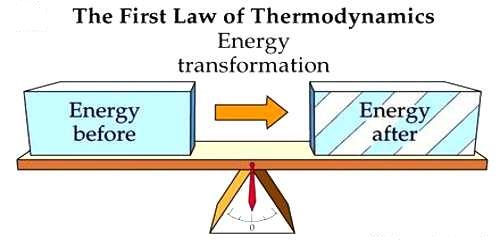Physics

# Principle of First Law of ThermodynamicsThe principle of First Law of Thermodynamics

Law: When work is transformed into heat or heat is transformed into work, then work and heat is directly proportional to each other.

Explanation: If Q amount of heat is produced due to the total transformation of W amount of work, then according to the first law of thermodynamics.

W ∞ Q or, W = JH

Here J is proportionality constant. It is called the mechanical equivalent of heat or Joule’s equivalent.

Thermodynamics is the branch of physics that deals with the relationships between heat and other forms of energy. The first law of thermodynamics is a special form of the principle of conservation of energy. The law of conservation of energy states that the total energy of an isolated system is constant; energy can be transformed from one form to another, but cannot be created or destroyed. Scientist Clausius expressed this law in general form. According to him, if heat energy is transformed into any other energy or any other energy is transformed into heat, then the total energy of the system remains same. Scientist Clausius defined the first law of thermodynamics as follows. It is called Clausius Principle.

Principle: When heat is supplied to any system or heat is absorbed by the system, then a part of this energy is used to increase the internal energy of the system and the rest energy is used to perform external work.

The first law makes use of the key concepts of internal energy, heat, and system work. It is used extensively in the discussion of heat engines. The standard unit for all these quantities would be the joule, although they are sometimes expressed in calories.